## Limits at Infinity

Limits at infinity are used to describe the behavior of functions as the independent variable increases or decreases without bound. If a function approaches a numerical value L in either of these situations, writeand f( x) is said to have a horizontal asymptote at y = L. A function may have different horizontal asymptotes in each direction, have a horizontal asymptote in one direction only, or have no horizontal asymptotes.

Evaluate 1: Evaluate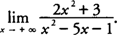Factor the largest power of x in the numerator from each term and the largest power of x in the denominator from each term.

You find that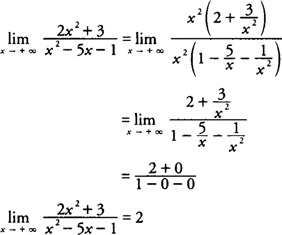The function has a horizontal asymptote at y = 2.

Example 2: Evaluate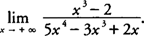Factor x 3 from each term in the numerator and x 4 from each term in the denominator, which yields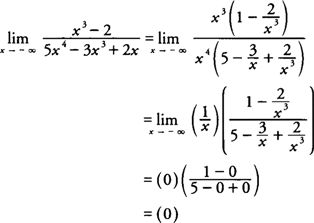The function has a horizontal asymptote at y = 0.

Example 3: Evaluate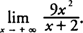.

Factor x 2 from each term in the numerator and x from each term in the denominator, which yieldsBecause this limit does not approach a real number value, the function has no horizontal asymptote as x increases without bound.

Example 4: Evaluate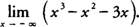.

Factor x 3 from each term of the expression, which yieldsAs in the previous example, this function has no horizontal asymptote as x decreases without bound.

Back to Top
A18ACD436D5A3997E3DA2573E3FD792A